Algebra of Matrices - Matrices and Determinants, Business Mathematics & Statistics

# Algebra of Matrices - Matrices and Determinants, Business Mathematics & Statistics Notes - B Com

## Document Description: Algebra of Matrices - Matrices and Determinants, Business Mathematics & Statistics for B Com 2022 is part of B Com preparation. The notes and questions for Algebra of Matrices - Matrices and Determinants, Business Mathematics & Statistics have been prepared according to the B Com exam syllabus. Information about Algebra of Matrices - Matrices and Determinants, Business Mathematics & Statistics covers topics like and Algebra of Matrices - Matrices and Determinants, Business Mathematics & Statistics Example, for B Com 2022 Exam. Find important definitions, questions, notes, meanings, examples, exercises and tests below for Algebra of Matrices - Matrices and Determinants, Business Mathematics & Statistics.

Introduction of Algebra of Matrices - Matrices and Determinants, Business Mathematics & Statistics in English is available as part of our B Com preparation & Algebra of Matrices - Matrices and Determinants, Business Mathematics & Statistics in Hindi for B Com courses. Download more important topics, notes, lectures and mock test series for B Com Exam by signing up for free. B Com: Algebra of Matrices - Matrices and Determinants, Business Mathematics & Statistics Notes - B Com
 1 Crore+ students have signed up on EduRev. Have you?

Addition and Subtraction of Matrices

Any two matrices can be added if they are of the same order and the resulting matrix is of the same order. If two matrices A and B are of the same order, they are said to be conformable for addition.

For example: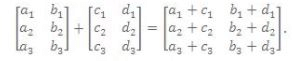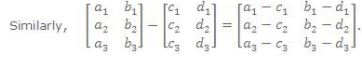Note:

• Only matrices of the same order can be added or subtracted.

• Addition of matrices is commutative as well as associative.

• Cancellation laws hold well in case of addition.

• The equation A + X = 0 has a unique solution in the set of all m × n matrices.

Scalar Multiplication

The matrix obtained by multiplying every element of a matrix A by a scalar λ is called the multiple of A by λ and its denoted by λ A i.e. if A = [aij] then λA = [λaij].

For example: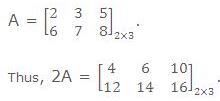Note: All the laws of ordinary algebra hold for the addition or subtraction of matrices and their multiplication by scalar.

Multiplication of Matrices

Two matrices can be multiplied only when the number of columns in the first, called the prefactor, is equal to the number of rows in the second, called the postfactor. Such matrices are said to be conformable for multiplication.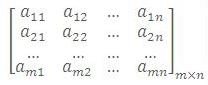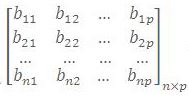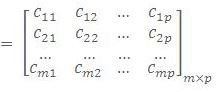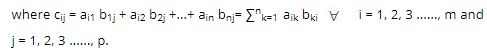Properties of Multiplication

• Matrix multiplication may or may not be commutative. i.e., AB may or may not be equal to BA

• If AB = BA, then matrices A and B are called Commutative Matrices.

• If AB = BA, then matrices A and B are called Anti-Commutative Matrices.

• Matrix multiplication is Associative

• Matrix multiplication is Distributive over Matrix Addition.

• Cancellation Laws not necessary hold in case of matrix multiplication i.e., if AB = AC => B = C even if A ≠ 0.

• AB = 0 i.e., Null Matrix, does not necessarily imply that either A or B is a null matrix.

Illustration: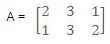and B =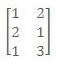show that AB ≠ BA.

Solution:

Here A.B =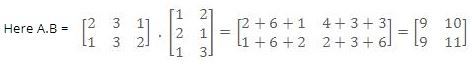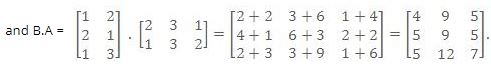Thus A.B ≠ B.A.

Illustration: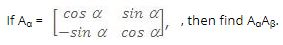Solution:

We have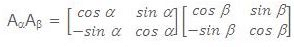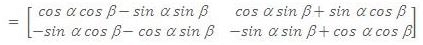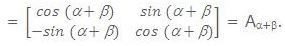At askIITians we provide you free study material on Set Theory and Functions and you get all the professional help needed to get through IIT JEE and AIEEE easily. AskIITians also provides live online IIT JEE preparation and coaching where you can attend our live online classes from your home!

The document Algebra of Matrices - Matrices and Determinants, Business Mathematics & Statistics Notes - B Com is a part of B Com category.
All you need of B Com at this link: B Com
 Use Code STAYHOME200 and get INR 200 additional OFF

Track your progress, build streaks, highlight & save important lessons and more!

,

,

,

,

,

,

,

,

,

,

,

,

,

,

,

,

,

,

,

,

,

,

,

,

;